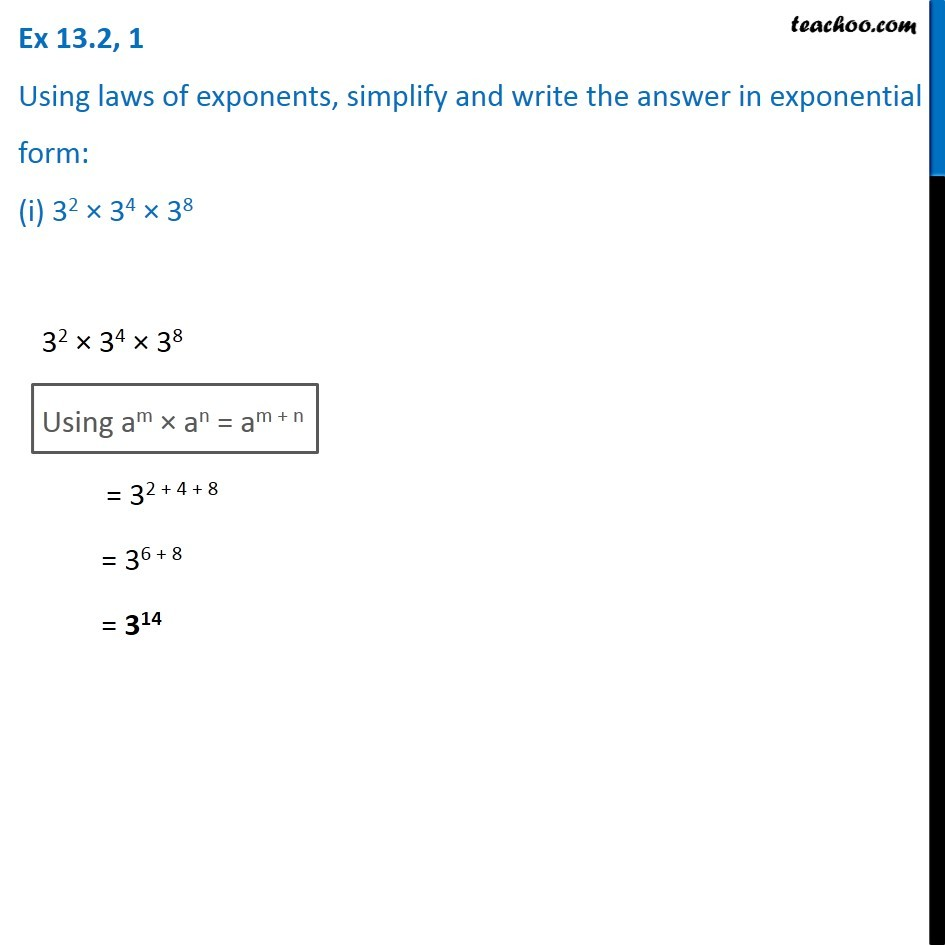1. Chapter 13 Class 7 Exponents and Powers
2. Concept wise
3. Law of exponents

Transcript

Ex 13.2, 1 Using laws of exponents, simplify and write the answer in exponential form: (i) 32 × 34 × 38 32 × 34 × 38 Using am × an = am + n = 32 + 4 + 8 = 36 + 8 = 314